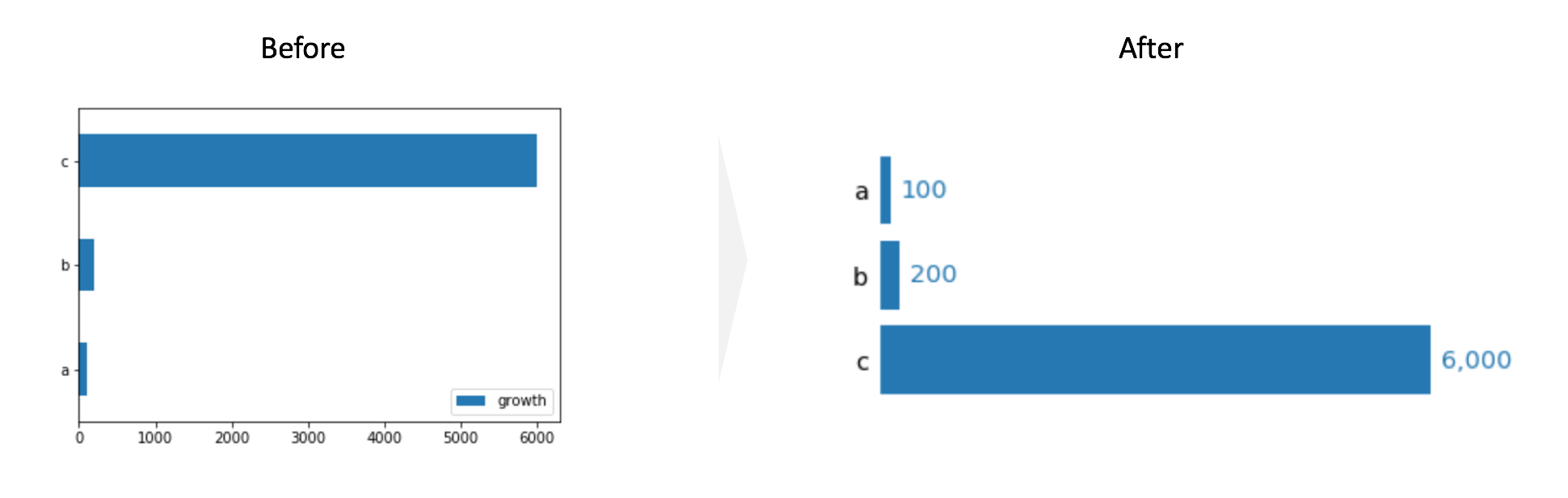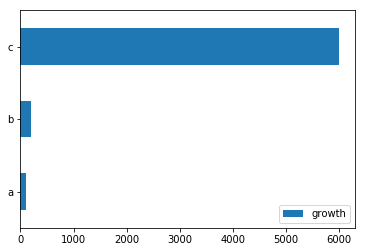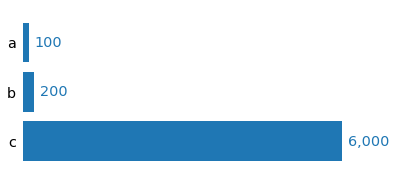# Minimalism visualization by matplotlib - Bar chart

## Overview¶

This post aims to simplify default bar chart by matpltlib as much as possible based on the philosophy of minimalist as below.## Prerequisite¶

In :
import matplotlib.pyplot as plt
import numpy as np
import pandas as pd
%matplotlib inline


## Bar plot¶

The key points are as follows:

1. Clarify the key message
2. Use comparison to show the size difference of data
3. Think of what is the most effective way to convey the message

### Default¶

In :
## Default
df_bar = pd.DataFrame([100, 200, 6000],
index=['a', 'b', 'c'],
columns=['growth'])
df_bar.plot(kind='barh');### Simplified Bar chart¶

• Delete legend
• Tighten the space between bars
• Re-order the y-axis
• Delete the square spines
• Delete ticks for x and y axis
• Delete tick label for x axis
• Increase the size of the label for y axis
• Display each value next to the bar
In :
# 1. Delete legend legend=False
# 2. Tighten the space between bars width=0.8
width = 0.8
fig, ax = plt.subplots(figsize=(6, 3))
df_bar.plot(kind='barh', legend=False, ax=ax, width=width);
# 3. Re-order the y-axis
ax.invert_yaxis()

# 4. Delete the square spines
[spine.set_visible(False) for spine in ax.spines.values()]

# 5. Delete ticks for x and y axis
# 6. Delete tick label for x axis
ax.tick_params(bottom=False, left=False, labelbottom=False)

# 7. Increase the size of the label for y axis
ax.tick_params(axis='y', labelsize='x-large')

# 8. Display each value next to the bar
vmax = df_bar['growth'].max()

for i, value in enumerate(df_bar['growth']):
ax.text(value+vmax*0.02, i, f'{value:,}', fontsize='x-large', va='center', color='C0')In :
## As a Function
def minimal_bar(series, ax=None, width=0.8, fisize=(6, 3),
reorder_yaxis=True, splines_off=True, delete_ticks=True, y_label_large=True, display_value=True):

if ax is None:
fig, ax = plt.subplots(figsize=fisize)

# 1. Delete legend legend=False
# 2. Tighten the space between bars width=0.8
series.plot(kind='barh', legend=False, ax=ax, width=width, color='C0');

# 3. Re-order the y-axis
if reorder_yaxis:
ax.invert_yaxis()

# 4. Delete the square spines
if splines_off:
[spine.set_visible(False) for spine in ax.spines.values()]

# 5. Delete ticks for x and y axis
# 6. Delete tick label for x axis
if delete_ticks:
ax.tick_params(bottom=False, left=False, labelbottom=False)

# 7. Increase the size of the label for y axis
if y_label_large:
ax.tick_params(axis='y', labelsize='x-large')

# 8. Display each value next to the bar
if display_value:
vmax = series.max()
for i, value in enumerate(series):
ax.text(value+vmax*0.02, i, f'{value:,}', fontsize='x-large', va='center', color='C0')

In :
minimal_bar(series=df_bar['growth'])